# GRE Subject Test: Math : Statistics

## Example Questions

1 2 3 5 Next →

### Example Question #7 : Range & Iqr

Find the range of: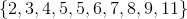Explanation:

Step 1: Locate the smallest and largest numbers in the set above...

Largest is, smallest isStep 2: To find the range, subtract smallest from largest...

Range=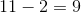### Example Question #1 : Range & Iqr

What is the range of the set,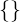?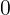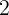Does Not Exist

Does Not Exist

Explanation:

Step 1: Define Range of a set..

You can calculate the range by subtracting the smallest number from the largest number in the set..

Step 2: There are no numbers in this set. This set is also known as the empty set..

The range of a set that has no elements does not exist.

### Example Question #1 : Range & Iqr

If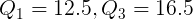, find the IQR (Inter-Quartile Range)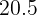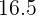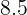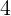Explanation:

Step 1: To find the Inter-Quartile Range, we need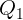and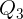.

The formula for the Inter-Quartile Range is: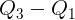.

Step 2: Plug in the given values of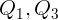to the equation...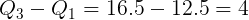So, the IQR=### Example Question #1 : Range & Iqr

Find the range: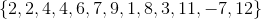Explanation:

Rearrange the numbers in the set from smallest to largest: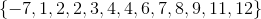To find the range, subtract the smallest number from the bigger number: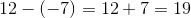The range is.

### Example Question #41 : Statistics

If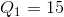and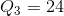, find the IQR.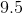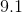Explanation:

The IQR (Inter-Quartile Range) is the distance from the third quartile and the first quartile. The IQR is always positive. Halfway betweenandis the Mean (or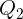.

To find the IQR, just subtractfrom.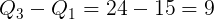### Example Question #42 : Other Topics

Find the range: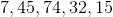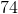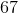Explanation:

The range is the difference between the largest and smallest number.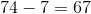1 2 3 5 Next →

### All GRE Subject Test: Math Resources